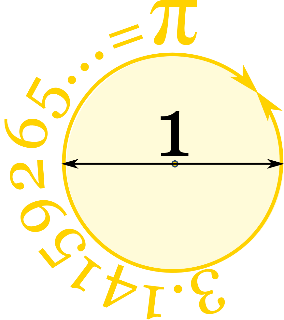# Can I Get Some Pi?

December 9, 2021

Pi is a mathematical constant. Although originally defined as the ration of a circle’s circumference to its diameter, it now has various equivalent definitions and appears in many formulas in all areas of mathematics and physics.

A circle’s diameter is the measurement from one side to another. The circumference is the measurement around the outside of the circle. If we draw a circle with a diameter of 1, then the circumference is 3.14159265...a number known as Pi. Pi is often written using the Greek symbol π.

Materials:

- 100 Toothpicks
- Large Poster Board
- Paper
- Writing Utensil
- Ruler

Procedure:
1. Using your ruler, measure the length of a toothpick and double it. Most toothpicks are 2.5 inches long – doubled is 5 inches! If your toothpick is a different length, double the length and use that number instead. This number is very important, so write it down for the next part.

2. Starting at one end of your poster board, use your ruler to

measure a space 5 inches (or your toothpick’s length

doubled) wide. Make a mark here and do the same all the way across your poster board. Once you have all of your marks, draw a line from the top of your poster board to the bottom at each mark. Your poster board should have lines from the top to the bottom with 5 inches of space between each one (or your toothpick’s length doubled).
3. Next, we are going to randomly dump our toothpicks onto the board. We want to make sure they are spread out and not clumped together. One way to make this easier is to put them all in a cup and pour them out onto the board.
4. Count how many toothpicks are touching or crossing the lines on your board.
5. Math time! We are going to use this formula to calculate a number that is close to pi.

6. The more times you do this experiment and look at the average of all of your trials, the closer to pi you will be.

7. To calculate the average from your trials, add all of your results together and then divide by the number of trials you did.

Explore more: Buffon's needle problem is one of the oldest in the field of geometric probability. It is a question that was first posed in the 18th century by French mathematician, Georges-Louis Leclerc, Conte de Buffon. The experiment demonstrates the relationship between a circle’s radius, diameter, circumference, and the value of pi. The radius is the measurement from the center of a circle to the side. The Law of Large Numbers states that as you increase the number of random trials of an experiment, the results will approach the theoretical expected value.